# Text-blocks

1. Problem and history

Let the text be divided in (not necessarily equal) passages of words, e.g. 100, 200, pagewise, sentencewise, etc. and the distribution of a chosen linguistic entity in these passages is sought. The passages can contain this entity zero times, once, twice,… . The variable x is thus the number of the given entity in that passage, and fx is the number of passages containing this entity x times. The origin of the reseach goes back to E. Zwirner and K. Zwirner (1935, 1938) who considered the distribution of different sounds in text-blocks and assumed the “law of small numbers” as the generating mechanism. Frumkina (1962) who probably did not know the work of Zwirners considered word occurrence as a “rare event” and applied automatically the Poisson distribution, Mosteller and Wallace (1964) derived the negative binomial distribution, Brainerd added the mixed Poisson distribution (1972a), Piotrowski, Bektaev, Piotrowskaja (1985) used the binomial distribution, some Russian authors used the normal distribution and Altmann, Burdinski (1982) who baptized this mechanism as Frumkina´s law derived the negative hypergeometric distribution which will be presented here. Leopold (1998) gives hints to other possible distributions. Köhler (2001) examined the distribution of syntactic constructions in text blocks. Best (2005) brought a general survey of results up to now. Piotrowski (1984) mentions the following applications of the text-block law:

(1) It can help to ascertain mechanically the membership of a word to a word class.

(2) It can help to identify terminologically or semantically dominant text units.

(3) It enables us to measure and ascertain the stylistic individuality of the text.

(4) It enables us to diagnostify the foci of some psychic deseases (cf. Paškovskij, Srebrjanskaja 1971).

(5) It helps to construct learning automata.

2. Hypothesis

The distribution of individual entities in text passages abides by the negative hypergeometric distribution.

3. Derivation (Altmann, Burdinski 1982)

Let the probability of a word A in language be p. This is Herdan´s (1956) assumption but as a matter of fact, fixed probabilities of language units are illusory. Nevertheless, this assumption can be used because p will be randomized. In a text passage in which A can occur maximally n times, the probability that it will occur exactly x times is given by the binomial distribution

(1)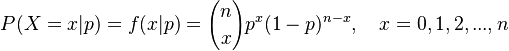$P(X=x|p) = f(x|p) = {n \choose x}p^x (1-p)^{n-x}, \quad x = 0,1,2,...,n$.

However, p is not constant since its value depends on the kind of text, on the length of the passage and especially on the environment (e.g. it cannot occur three times one behind the other). Thus the probability of its occurrence in an individual position of the passage is a variable with its own distribution.

Altmann and Burdinski (1982) assumed that p has a beta distribution given as

(2)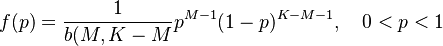$f(p) = \frac{1}{b(M,K-M}p^{M-1}(1-p)^{K-M-1}, \quad 0,

where B(.) is the beta function (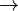$\rightarrow$ Appendix). The common distribution of x and p is now

(3)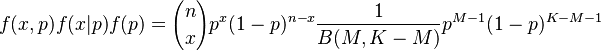$f(x,p) f(x|p)f(p) = {n \choose x}p^x (1-p) ^{n-x} \frac{1}{B(M,K-M)} p^{M-1} (1-p) ^{K-M-1}$

which can be solved for x by integrating (3) according to p. As a result we obtain

(4)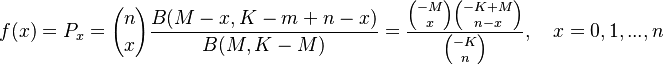$f(x) = P_x = {n \choose x}\frac{B(M-x, K-m + n-x)}{B(M,K-M)} = \frac{{-M \choose x}{-K+M \choose n-x}}{{-K \choose n}}, \quad x= 0,1,...,n$

i.e. the negative hypergeometric distribution. Here M, N, n are parameters. It can easily be shown that the other distributions mentioned above are limiting cases of the negative hypergeometric:

(i) when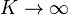$K\rightarrow \infty$,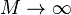$M\rightarrow \infty$,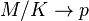$M/K\rightarrow p$ then the negative hypergeometric distribution converges to the binomial distribution (Piotrowski et al. version) (see (1));

(ii) when$K\rightarrow \infty$,$M\rightarrow \infty$,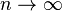$n\rightarrow \infty$,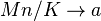$Mn/K\rightarrow a$ then the negative hypergeometric distribution converges to the Poisson distribution (Brainerd´s version):$P_x = \frac{a^x e^{-a}}{x!}, \quad x=0,1,2,...:\quad a>0$

(iii) when$K\rightarrow \infty$,$n\rightarrow \infty$,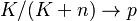$K/(K+n)\rightarrow p$ then the negative hypergeometric distribution converges to the negative binomial distribution (Mosteller-Wallace´ version):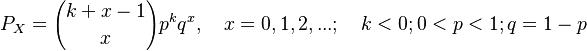$P_X = {k+x-1 \choose x}p^k q^x, \quad x= 0,1,2,...;\quad k<0;0

Thus each of the above models has its partial justification. The normal distribution is not taken into account since it is continuous but the convergence to it can easily be shown.

Example: Distribution of nouns in text blocks

Piotrowski, Bektaev, Piotrowskaja (1985) examined the distribution of nouns in passages in Auezov´s novel “Put´ Abaja” and found the frequencies given in Table 1 to which they fitted the binomial distribution. In the last column the negative hypergeometric distribution is shown. Since there are no passages without nouns, both theoretical distributions are 1-displaced.

In both cases the fitting is satisfactory, the negative hypergeometric is somewhat better.

Fig 1. Fitting the binomial distribution to data of Piotrowski et al. (1985)

Fig. 2. Fitting the negative hypergeometric distribution to data of Piotrowski et al. (1985)

Example: Distribution of the Russian preposition “bez” in text blocks

Frumkina (1962) examined the occurrence of the Russian preposition “bez” in 110 passages consisting of 1000 words each from texts by Pushkin and fitted the Poisson distribution. In the last column of Table 2 the negative hypergeometric distribution is shown, too.

Fig. 3. Fitting the Poisson distribution to Frumkina´s data

Fig. 4. Fitting the negative hypergeometric distribution to Frumkina´s data

Example: Distribution of the article “das” in German text blocks

Altmann and Burdinski (1982) examined the occurrence of the German article “das” in nominative in passages from S. Lenz “Deutschstunde”. They fitted the negative hypergeo-metric distribution changing stepwise n and showed the gradual convergence to the negative binomial distribution (cf. Table 3)

As can be seen in Table 3, all fittings are good and improve with increasing n and K. This is a sign of convergence to the negative binomial distribution which, as a matter of fact, shows the best result.

Fig. 5. Fitting the negative binomial distribution to data of Lenz

Example. Distribution of the indirect object (Köhler 2001)

Köhler (2001) analyzed syntactic constructions in text blocks e.g. participle clauses, relative clauses, infinitival clauses, prepositional objects, indirect objects, logical direct objects and stated that all follow the negative binomial distribution. The fitting of this distribution to the Susanne Corpus (Sampson 1995) to the number of blocks with x occurrences of indirect object is shown in Table 4 and Fig. 6.

Further investigations have been carried out on letters (Schulte 2002, Suhren 2002), grammatical and lexical words (Best 2001, ²2003; Billmeier 1968, Muller 1972; Suhren 2002), semantic groups of words (Muller 1972) and groups consisting of 3 words (Piotrowski, Bektaev, Piotrowskaja 1985). They all abide by the law of text blocks, too (Best 2005). Knauer (1955: 146) yields the proportion of vowels in text-blocks of 100 phones in French and Italian.

4. Authors: U. Strauss, G. Altmann, K.-H. Best

5. References

Altmann, G. (1988a). Wiederholungen in Texten. Bochum, Brockmeyer.

Altmann, G., Burdinski, V. (1982). Towards a law of word repetitions in text-blocks. Glottometrika 4, 147-167.

Bektaev, K.B., Lukjanenkov (1971). O zakonach raspredelenija edinic pis'mennoj reči. In: Piotrowski, R.G. (ed.), Statistika reči i avtomatičeskij analiz teksta: 47-112. Leningrad: Nauka.

Best, K.-H. (2005). Sprachliche Einheiten in Textblöcken. Glottometrics 9, 1-12.

Best, K.-H. (2006). Quantitative Untersuchungen zum Niederdeutschen und Niederländischen. Göttinger Beiträge zur Sprachwissenschaft 13, 51-71.

Best, K.-H. (2006). Quantitative Linguistik. Eine Annäherung. 3., stark überarbeitete und ergänzte Auflage. Göttingen: Peust & Gutschmidt.

Billmeier, G.. (1968). Über die Signifikanz von Auswahltexten. Untersuchung auf der Grundlage von Zeitungstexten. In: Moser, Hugo u.a. (Hrsg.), Forschungsberichte des Instituts für deutsche Sprache 2, 126-171.

Brainerd, B. (1972a). Article use as an indirect indicator of style among English-language authors. In: Jäger, S. (ed.), Linguistik und Statistik: 11-32. Braunschweig, Vieweg.

Frumkina, R.M. (1962). O zakonach raspredelenija slov i klassov slov. In: Mološnaja, T.N. (ed.), Strukturno-tipologičeskie issledovanija: 124-133. Moskva: ANSSSR.

Herdan, G. (1956). Language as Choice and Chance. Groningen: Nordhoff.

Knauer, K. (1955). Grundfragen einer mathematischen Stilistik. Forschungen und Fortschritte 29, 140-149.

Köhler, R. (2001). The distribution of some syntactic construction types in text blocks. In Uhlířova, L., Wimmer, G., Altmann, G., Köhler, R. (Eds.), Text as a linguistic paradigm: levels, constituents, constructs. Festschrift in honour of Ludek Hřebíček: 136-148. Trier: WVT.

Leopold, E. (1998). Stochastische Modellierung lexikalischer Evolutionsprozesse. Hamburg: Kovač.

Maškina, L.E. (1968). O statističeskich metodach issledovanija leksiko-grammatičeskoj distribucii. Minsk, Diss.

Morton, A.Q., Levison, M. (1966). Some indicators of authorship in Greek prose. In: Leed, J. (ed.), The computer and literary style: 141-179. Kent, Ohio: Kent State UP.

Mosteller, F., Wallace, D.L. (1964). Inference and disputed authorship: The Federalist. Reading, Mass, Addison-Wesley.

Muller, Ch. (1972). Einführung in die Sprachstatistik. München: Hueber.

Paškovskij, V.E., Srebrjanskaja, I.I. (1971). Statističeskie ocenki pis'mennoj reči bol'nych šizofreniej. In: Inženernaja lingvistika. Leningrad.

Piotrowski, R.G. (1984). Text – Computer – Mensch. Bochum: Brockmeyer.

Piotrowski, R.G., Bektaev, K.B., Piotrowskaja, A.A. (1985). Mathematische Linguistik. Bochum, Brockmeyer.

Suhren, S. (2002). Untersuchung zum Gesetz von Zwirner, Zwirner und Frumkina am Beispiel des niederdeutschen „De lütte Prinz“. Staatsexamensarbeit, Göttingen.

Zwirner, E., Ezawa, K. (Hrsg.) (1966, 1968, 1969). Phonometrie, Erster-Dritter Teil. Basel/ New York: Karger.

Zwirner, E., Zwirner, K. (1935). Lauthäufigkeit und Zufallsgesetz. Forschungen und Fortschritte 11, Nr. 4: 43-45. (Also in: Zwirner & Ezawa (Hrsg.), Dritter Teil: 55-59.)

Zwirner, E., Zwirner, K. (1938). Lauthäufigkeit und Sprachvergleichung. Monatsschrift für höhere Schulen 37: 246-253. (Also in: Zwirner & Ezawa (Hrsg.), Dritter Teil, 68-74.)